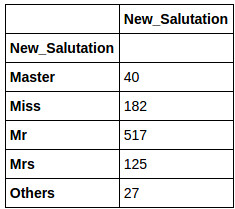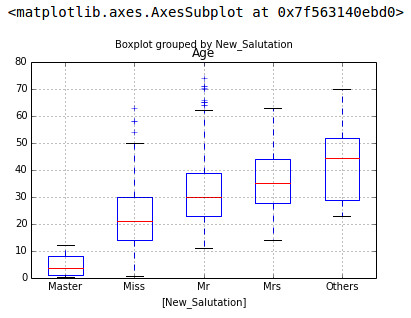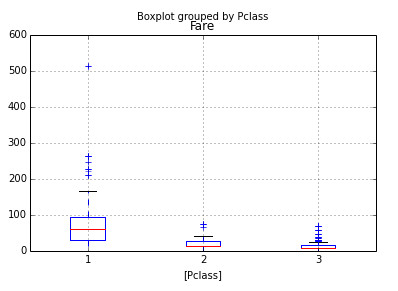Home » Data Munging in Python (using Pandas) – Baby steps in Python

# Data Munging in Python (using Pandas) – Baby steps in Python

Time flies by! I see Jenika (my daughter) running around in the entire house and my office now. She still slips and trips – but is now independent to explore the world and figure out new stuff on her own. I hope I would have been able to inspire similar confidence with use of Python for data analysis in the followers of this series.

For those, who have been following, here are a pair of shoes for you to start running!By end of this tutorial, you will also have all the tools necessary to perform any data analysis by yourself using Python.

### Recap – Getting the basics right

In the previous posts in this series, we had downloaded and setup a Python installation, got introduced to several useful libraries and data structures and finally started with an exploratory analysis in Python (using Pandas).

In this Pandas tutorial, we will continue our journey from where we left it in our last tutorial – we have a reasonable idea about the characteristics of the dataset we are working on. If you have not gone through the previous article in the series, kindly do so before proceeding further.

### Data munging – recap of the need

While our exploration of the data, we found a few problems in the dataset, which need to be solved before the data is ready for a good model. This exercise is typically referred as “Data Munging”. Here are the problems, we are already aware of:

1. About 31% (277 out of 891) of values in Age are missing. We expect age to play an important role and hence would want to estimate this in some manner.
2. While looking at the distributions, we saw that Fare seemed to contain extreme values at either end – a few tickets were probably provided free or contained data entry error. On the other hand \$512 sounds like a very high fare for booking a ticket

In addition to these problems with numerical fields, we should also look at the non-numerical fields i.e. Name, Ticket and Cabin to see, if they contain any useful information.

#### Check missing values in the dataset

Let us look at Cabin to start with. First glance at the variable leaves us with an impression that there are too many NaNs in the dataset. So, let us check the number of nulls / NaNs in the dataset

`sum(df['Cabin'].isnull()) `

This Pandas command should tell us the number of missing values as isnull() returns 1, if the value is null. The output is 687 – which is a lot of missing values. So, we’ll need to drop this variable.

Next, let us look at variable Ticket. Ticket looks to have mix of numbers and text and doesn’t seem to contain any information, so will drop Ticket as well.

`df = df.drop(['Ticket','Cabin'], axis=1) `

#### How to fill missing values in Age using Pandas fillna?

There are numerous ways to fill the missing values of Age – the simplest being replacement by mean, which can be done by following code:

```meanAge = np.mean(df.Age)
df.Age = df.Age.fillna(meanAge)```

The other extreme could be to build a supervised learning model to predict age on the basis of other variables and then use age along with other variables to predict survival.

Since, the purpose of this tutorial is to bring out the steps in data munging, I’ll rather take an approach, which lies some where in between these 2 extremes. The key hypothesis is that the salutations in Name, Gender and Pclass combined can provide us with information required to fill in the missing values to a large extent.

Here are the steps required to work on this hypothesis:

##### Step 1: Extracting salutations from Name

Let us define a function, which extracts the salutation from a Name written in this format:

Family_Name, Salutation. First Name

```def name_extract(word):
return word.split(',').split('.').strip()
```

This function takes a Name, splits it by a comma (,), then splits it by a dot(.) and removes the whitespaces. The output of calling function with ‘Jain, Mr. Kunal’ would be Mr and ‘Jain, Miss. Jenika’ would be Miss

Next, we apply this function to the entire column using apply() function and convert the outcome to a new Pandas DataFrame df2:

```df2 = pd.DataFrame({'Salutation':df['Name'].apply(name_extract)})
```

Once we have the Salutations, let us look at their distribution. We use the good old groupby after merging the DataFrame df2 with DataFrame df:

```df = pd.merge(df, df2, left_index = True, right_index = True) # merges on index
temp1 = df.groupby('Salutation').PassengerId.count()
print temp1
```

Following is the output:

```Salutation
Capt          1
Col           2
Don           1
Dr            7
Jonkheer      1
Major         2
Master       40
Miss        182
Mlle          2
Mme           1
Mr          517
Mrs         125
Ms            1
Rev           6
Sir           1
the Countess  1
dtype: int64

```

As you can see, there are 4 main Salutations – Mr, Mrs, Miss and Master – all other are less in number. Hence, we will combine all the remaining salutations under a single salutation – Others. In order to do so, we take the same approach, as we did to extract Salutation – define a function, apply it to a new column, store the outcome in a new Pandas DataFrame and then merge it with old DataFrame:

```def group_salutation(old_salutation):
if old_salutation == 'Mr':
return('Mr')
else:
if old_salutation == 'Mrs':
return('Mrs')
else:
if old_salutation == 'Master':
return('Master')
else:
if old_salutation == 'Miss':
return('Miss')
else:
return('Others')
df3 = pd.DataFrame({'New_Salutation':df['Salutation'].apply(group_salutation)})
df = pd.merge(df, df3, left_index = True, right_index = True)
temp1 = df3.groupby('New_Salutation').count()
temp1
df.boxplot(column='Age', by = 'New_Salutation')
```

Following is the outcome for Distribution of New_Salutation and variation of Age across them:##### Step 2: Creating a simple grid (Class x Gender) x Salutation

Similarly plotting the distribution of age by Sex & Class shows a sloping:So, we create a Pivot table, which provides us median values for all the cells mentioned above. Next, we define a function, which returns the values of these cells and apply it to fill the missing values of age:

```table = df.pivot_table(values='Age', index=['New_Salutation'], columns=['Pclass', 'Sex'], aggfunc=np.median)
# Define function to return value of this pivot_table
def fage(x):
return table[x['Pclass']][x['Sex']][x['New_Salutation']]
# Replace missing values
df['Age'].fillna(df[df['Age'].isnull()].apply(fage, axis=1), inplace=True)
```

This should provide you a good way to impute missing values of Age.

#### How to treat for Outliers in distribution of Fare?

Next, let us look at distribution of Fare by Pclass:As expected the means of Fare line up neatly by Pclass. However, there are a few extreme values. One particular data point which grabs attention, the fare of 512 for Class 1 – this looks like a likely error. Again there are multiple ways to impute this data – replace by mean / median of Class 1 or you can also replace the value by the second highest value, which is closer to other data points.

You can choose you pick and replace the values. The commands are similar to the ones mentioned above.

#### End Notes:

Now our dataset is ready for building predictive models. In the next tutorial in this series, we will try out various predictive modeling techniques to predict survival of Titanic passengers and compare them to find out the best technique.

A quick recap, by now – you would be comfortable performing exploratory analysis and Data Munging in Pandas. Some of the steps in this tutorial can feel overdone for this problem – the idea was to provide a tutorial, which can help you even on bigger problems. If you have any other tricks, which you use for data cleaning and munging, please feel free to share them through comments below.

You can also read this article on our Mobile APP•pascal says:

quick question about the code snippet where you extract salutations and combine all except the major four into the ‘others’ category: is there a reason why you aren’t using ‘elif:’ instead of repeating
‘else:
if:’
?

thanks!

•Kunal Jain says:

No particular reason. I guess else if comes more intuitively because of coding in other languages, so I normally stick with it.

•guindilla says:

Cheers,

From a conceptual point of view, what is the interest of putting all other salutations under “other”? I believe we can get more information by explicitely managing them. For instance, Mlle corresponds to Miss, Mme corresponds to Mrs, Don corresponds to Mr (all those because of different languages). Furthermore, we could say that Sir, Countess, Col, Jonkheer, etc. could be substituted by Mr, Mrs or Miss depending on the context. Is this because we can modify our train data but not the test data, and thus we would not be able to extrapolate our conclusions?

Thanks.

•Allison Minugh says:

You raise a good point, and, for me, this is why content expertise is a must for data management–even if we are just classifying salutations. Imagine the difference it makes in other cases! Your examples would provide more accurate, useful data.

•Kunal Jain says:

Guindilla / Allison,

You raise a very good point here. Some of these Salutations could have been merged into the 4 main salutations, Some of them would still remain in Others category – like Dr. Also, I wasn’t sure of determining Gender basis the first names and we would need to keep an Others category irrespective, just in case train dataset has any other designation.

But your point is completely spot on – these transformation could increase the accuracy of imputation.

Regards,
Kunal

•Hassine Saidane says:

Here is the link to an interesting development in data analysis worth a read. Hope you’ll enjoy and cogitate upon:

LET THE DATA ASK THE QUESTIONS.

•Benjamin says:

Thanks for the article.
I have noticed a minor errata. The missing values for Age aren’t 277 but 177: (891 – 714) or following your article: sum(df[‘Age’].isnull())

•Vaishali says:

Thanks for your post. Just going to add some praise to the pile. It’s easy to go find some documentation and nut things out eventually with a cool new tool/package but after finding your post I’ve been coming back here on the regular for a concise explanation and example of these fundamental operations in pandas, super clear and it made getting up and running easy. Thank you.

•Sandeep says:

Hello Kunal,

great article, for a newbie like me in Python, its invaluable..
I liked the pivot table approach to impute…I was wondering if you could write an article showing how to use a ‘knn’ / ‘random-forest’ method to impute values…
mean/median is fine on a small data set but on large data sets with many outliers and missing values, I think it’s not a good approach to fill 40-50% values in a column with median/mean..

i googled but couldnt find a good tutorial / link explaining how to do it in Python..only think I could find is the scitkit github page where its an open feature to be added for ‘knn’ imputation in sklearn.preprocessing import Imputer

•kkndgit says:

Hi Kunal
great artical, thanks a lot.
but I have a question about the lookup values from a pivot table by a pd dataframe, as you did in fillna in ‘age’.
the function “fage”,how dose it work? the x[Pclass] in the fage, what does that mean? Where and which key word can help me to google the question out? thanks!

•MichaelReinhar9 says:

I had another problem getting the code to work in one place, the place that kkndgit is talking about. When I tried to run the code:

df[‘Age’].fillna(df[df[‘Age’].isnull()].apply(fage, axis=1), inplace=True)

I got the error:

ValueError: invalid fill value with a

Did anyone else have this problem? Maybe something has changed since last September? I tried adding ‘pd.’ to a bunch of things but that didn’t work. Any other suggestions?

Thanks again for these great tutorials.

•Robin says:

What is the link to the next tutorial/article in the series? the one where predictive modelling will be discussed on the titanic data set